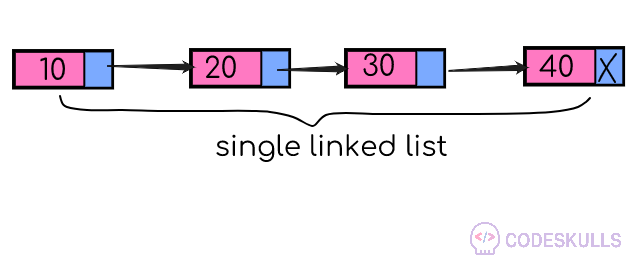# Create and Traverse a Singly Linked List

1. create a node template with the basic code given belowstruct sll {
int data;
struct node *next;
};

data is the variable, where we can store data of that particular node

*next is the node pointer which has the address of next node

use typedef keyword to create an alias name for structure sll (data type).here in the below code new datatype known as node is created using the typedef keyword

2.decalre a new pointer *head to the structure node, we can use this head pointer to access the data from all nodes, assign this pointer as value NULL

3.write a function for creating a newnode using malloc function and taking node data value from the user with in the function

node* getnode()
{
node * newnode;
newnode = (node *) malloc(sizeof(node));
printf("\n Enter data: ");
scanf("%d", &newnode -> data);
newnode -> next = NULL;
return newnode;
}

In the above code getnode() function returns the node type of pointer ,make sure that the next pointer of the newnode points to null (newnode -> next = NULL), return the newnode from the function .when the getnode function is called in the program this creates a node in the memory.

4.create two more pointers known as *newnode,*temp inside createnodes function, use FOR loop for condition (i<no of nodes).

call getnode( ) function for the newnode pointer

newnode = getnode();

create a if block for condtion (head==NULL) then assign newnode to the head

for the else block

• assign temp=head (for *temp temporary node, to access all data in the nodes)
• use while condition temp->next!=NULL (to exit the while loop when temp pointer encounters NULL, single linked list has last elements next address is NULL, so the while stops here, now
• replace next value of current temp node using temp->next
• at last temp pointer is at last node now assigin newnode to the last node using temp->next=newnode
while(temp -> next != NULL)
temp = temp -> next;
temp -> next = newnode;

### Traverse a Singly Linked List#

1. create a temporary pointer *temp, assign head as a reference to it (temp=head)
2. create a while condition (temp!=NULL)
3. in while condition, print (temp->data) because every node has data in its data field
4. now change temp value to data present address in temp-> next, (next node)printf("%d-->", temp -> data);
temp = temp -> next;

#### Example program to create and traverse a linked list#

# include <stdio.h>
# include <conio.h>
# include <stdlib.h>
{
int data;
};
node* getnode()
{
node * newnode;
newnode = (node *) malloc(sizeof(node));
printf("\n Enter data: ");
scanf("%d", &newnode -> data);
newnode -> next = NULL;
return newnode;
}
void createlist(int n)
{
int i;
node *newnode;
node *temp;
for(i = 0; i < n; i++)
{
newnode = getnode();
{
}
else
{
while(temp -> next != NULL)
temp = temp -> next;
temp -> next = newnode;
}
}
}
void traverse()
{
node *temp;
printf("\n The contents of List : \n");
{
printf("\n Empty List");
return;
}
else
{
while(temp != NULL)
{
printf("%d-->", temp -> data);
temp = temp -> next;
}
}
printf(" X ");
}
int main()
{
int n;
printf("Enter the total number of nodes: ");
scanf("%d", &n);
createlist(n);
printf("\nData in the list \n");
traverse();
}

OUTPUT:

Enter the total number of nodes: 5
Enter data: 11
Enter data: 22
Enter data: 33
Enter data: 44
Enter data: 55
Data in the list
The contents of List:
11-->22-->33-->44-->55--> X
Last updated on by vishal devxo